# Henkin construction

(diff) ← Older revision | Latest revision (diff) | Newer revision → (diff)

The method of constants was introduced by L. Henkin in 1949 [a1] to establish the strong completeness of first-order logic (cf. Completeness (in logic)). Whilst this method originally involved the deductive apparatus of first-order logic, it can be modified so as to employ only model-theoretic ideas (cf. Model (in logic); Model theory).

Letbe a first-order logical language with equality, and consider a set of sentences inwhich is finitely satisfiable in the sense that each of its finite subsets has a model. Since the collection of finitely satisfiable sets is closed under unions of chains, each such set can be extended to one which is maximal in the sense that it is finitely satisfiable and contains every sentence inor its negation. Whencontains constant terms, each maximal set ininduces an equivalence relation on the set of constant terms: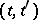is in this relation provided that the equation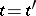is a member of the maximal set. Letdenote the equivalence class of. An interpretation forcan be constructed on the partition induced by this relation. On this interpretation, each individual constant in the non-logical vocabulary ofdenotes its equivalence class;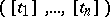is in the extension of the-ary predicateif and only if the sentence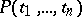is a member of the maximal set; andis in the extension of the-ary functional constantif and only if the equationis a member of the maximal set. This interpretation is a model of the maximal set if the set is term-complete in the sense that it contains an instance of each existential sentence it contains. This interpretation is called a Henkin model for the maximal and term-complete set.

Not every first-order language contains constant terms. And even whencontains constant terms, there are finitely satisfiable sets inwhich cannot be extended to maximal and term-complete sets in. In such cases the Henkin construction proceeds by adding new constants to the non-logical vocabulary ofin such a way that the finitely satisfiable set incan be extended to a maximal and term-complete set in the extended language.

H.J. Keisler [a2] modified the Henkin construction at the point where the new constants are introduced. Letdenote the collection of finite subsets of a finitely satisfiable set. For each member of, choose a model of that set.is the family (indexed by) of such models. Expand the non-logical vocabulary ofby adding the members of the direct product of the domains of the members ofas individual constants. Members of this direct product are functions onwhose value at each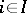is a member of the domain of theth member of. Theth member ofis expanded to interpretations of the extended language by having each function in the direct product denote its value at. Let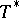denote the resulting family of interpretations. The theory ofis the set of all sentences in the extended language true on all members of. The union of the theory ofand the finitely satisfiable set fromis itself finitely satisfiable, and any maximal extension of this union is term complete. The Henkin model for such a maximal extension is called a Henkin–Keisler model.

Generalizing the above, letbe any non-empty set and letbe a family of interpretations forindexed by. As above, expand the non-logical vocabulary ofby adding the direct product of the domains of the members ofas individual constants, expand the members ofto interpretations of the extended language as above, and letdenote the resulting family of interpretations. The theory ofis finitely satisfiable and any maximal extension of this set is term complete.

Henkin–Keisler models can be seen as both a specialization of the Henkin construction and as an alternative to the ultraproduct construction (cf. also Ultrafilter). There is a natural correspondence between maximal extensions of the theory ofand ultrafilters (cf. Ultrafilter) on. Associate with each sentence in the expanded language the set of indices (from) of those members ofon which the sentence is true. Given an ultrafilter on, consider the set of sentences in the extended language whose associated set of indices is a member of the ultrafilter. This set is a maximal extension of the theory of. Further, if all members ofare non-trivial in the sense that their domains contain at least two objects, then given any maximal extension of the theory of, the collection of sets of indices associated with the members of the maximal extension is an ultrafilter on. Finally, the Henkin–Keisler model of any maximal extension of the theory of, when restricted to, is isomorphic to the ultraproduct of the members ofover the corresponding ultrafilter.

How to Cite This Entry:
Henkin construction. Encyclopedia of Mathematics. URL: http://encyclopediaofmath.org/index.php?title=Henkin_construction&oldid=13415
This article was adapted from an original article by G. Weaver (originator), which appeared in Encyclopedia of Mathematics - ISBN 1402006098. See original article